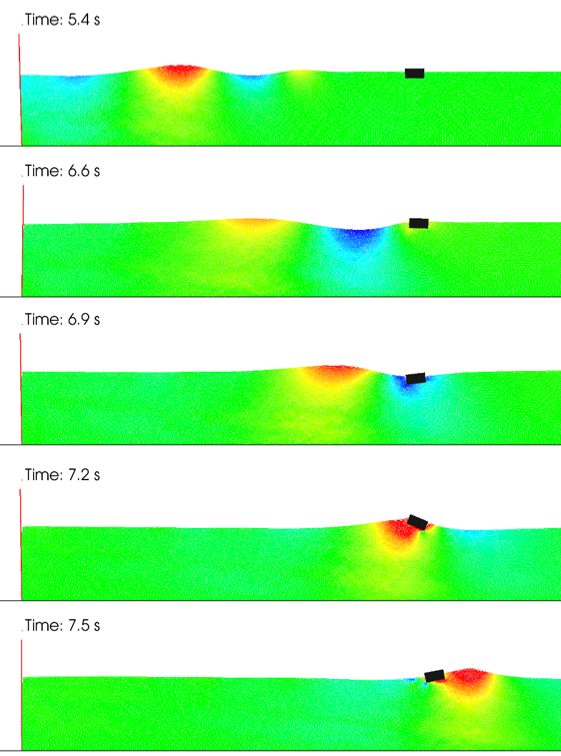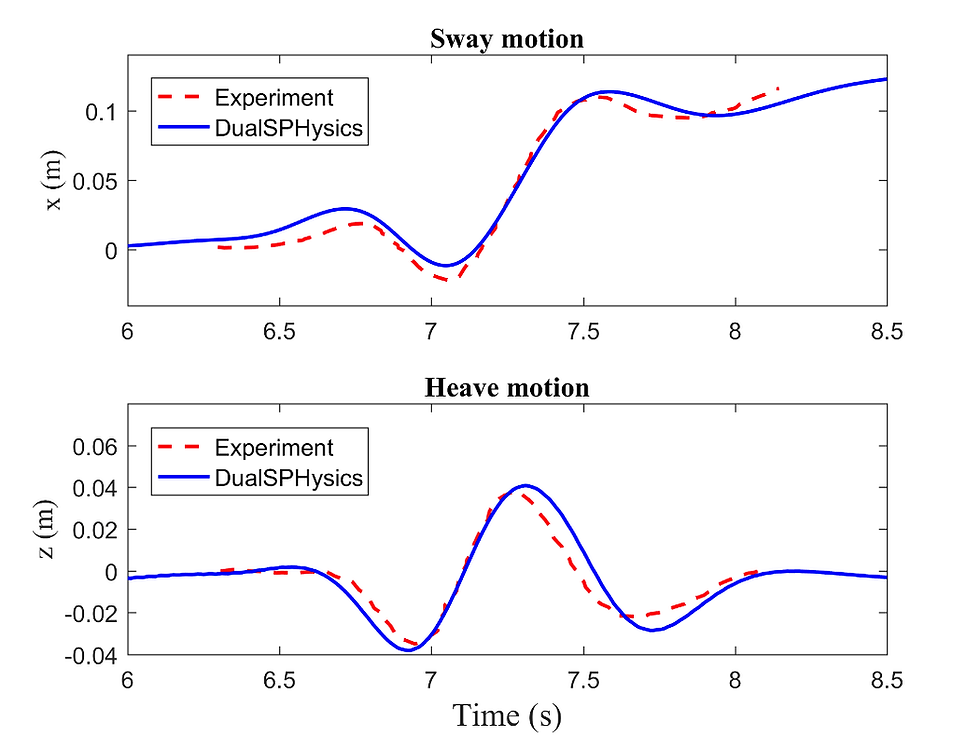top of page

## Test 12

2-D Wave interaction with floating body

(A. Crespo, A. Colagrossi)

### Introduction

The test proposed here is a good benchmark to validate the interaction of the fluid with rigid floating bodies and the correct buoyancy of the objects. Hence, the study of a floating body subjected to a wave packet is validated with experimental data. At the focusing point the wave packet is quite steep and has a height equal to the body. Then, a nonlinear behaviour is observed in both the resulting wave evolution and the body motion.

### Flow phenomena

Buoyancy, nonlinear waves. Measure of motion and rotation of a rigid body.

### Geometry

The setup of the experiment is shown in the figure below. The floating body is a rectangular prism 10 cm long, 5 cm high and 29 cm wide, with density relative to water being 0.68. The mass of the body (aluminum box) is 0.986 kg in 3D and the moment of inertia is 14 kg cm^2, but the simulation is performed in 2D. The black line is the flap wavemaker that follows a prescribed motion (see “Boundary Conditions” section). The center of the box is at 2.11 m from the flap. The experiment is fully described in Hǎdzić et al. (2005) and Xing-Kaeding (2006).Figure 1. Set-up of the experiment described in Hǎdzić et al. (2005).

### Boundary conditions

The file Flap.dat contains two columns which are respectively: time and degrees of rotation of the flap. The axis of rotation of the flap is its position at the bottom. The figure shows the time history of the flap wavemaker angle used to generate the wave packet.Figure 2. Time series of the flap wavemaker angle.

### Initial conditions

Gravity is acting on the fluid. The geometry initial conditions are reported in the “Geometry” section.

### Results specification

During the experiments the surface elevation was recorded by two fixed probes; one before the location of the body (x=1.16m) and other after the body (x=2.66m). In addition, the time histories of the 2-D floating body motions (sway and heave) and the 2-D rotation (roll angle) were also measured.

### Results format

The time histories of surface elevation (Probe_N1 and Probe_N2), the floating body motions (Heave and Sway) and the rotation (Roll) are available in the ASCII files: Elevation.dat, Motions.dat, Rotation.dat. Elevation.dat: Column 1=Time(s), 2=Probe_N1(m), Column 3=Time(s), 4=Probe_N2(m) Motions.dat: Column 1=Time(s), 2=Heave_N1(m), Column 3=Time(s), 4=Sway(m) Rotation.dat: Column 1=Time(s), 2=Roll(degrees).

### Benchmark results

The present test has been solved with the open-source DualSPHysics. Different instants of simulation can be seen in Figure 3.Figure 3. Different instants of the simulation using DualSPHysics. Colour represents the horizontal velocity of the fluid).

1) Surface elevation at two locations; one before of the body (x=1.16m) and other after the body (x=2.66m).Figure 4. Time series of experimental and numerical free-surface surface elevation.

2) Floating body motions.Figure 5. Experimental and numerical time series of the floating body motions (heave and sway).

### Publications using this test case as a benchmark

• Bouscasse, B., Colagrossi, A., Marrone, S., Antuono, M. “Nonlinear water wave interaction with floating bodies in SPH”, Journal of Fluids and Structures, 42: 112-129, 2013.

• Barreiro A, Crespo AJC, Domínguez JM, García-Feal O, Zabala I, Gómez-Gesteira M. “Quasi-Static Mooring solver implemented in SPH”, Journal of Ocean Engineering and Marine Energy, 2(3): 381-396, 2016. doi: 10.1007/s40722-016-0061-7.

### References

• Hǎdzić I, Hennig J, Peric M, Xing-Kaeding Y. “Computation of flow-induced motion of floating bodies”, Applied Mathematical Modelling, 29: 1196–1210, 2005.

• Xing-Kaeding, Y. “Unified approach to ship seakeeping and maneuvering by a RANSE method”. PhD Thesis, 2006.

bottom of page